# Ray Optics and Optical Instrument - Reversibility of Light

Law of Reversibility: When the ray of light reverse back to its own path after refraction and reflection, this phenomenon is called Reversibility.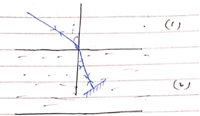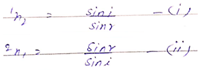Multiply both equation.

1n2 X 2n1 = 1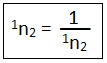When the light ray passes though multi medium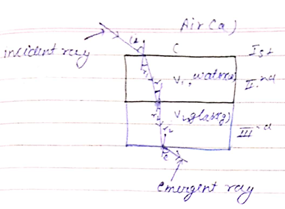At 1st Interface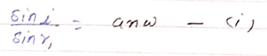At 2nd Interface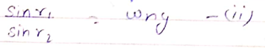At 3rd interface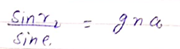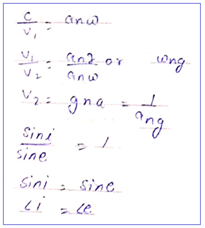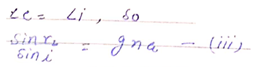Multiplying equation (i), (ii) & (iii)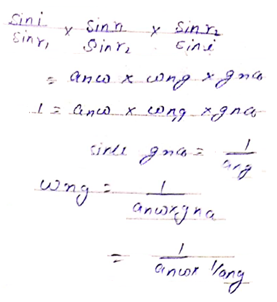Apparent depth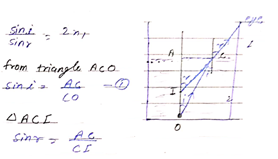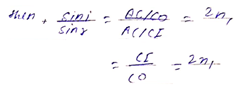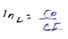If C is very close to A, then OC = OA = real and IC = IA = Apparent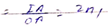2n1 = Apparent Depth / Real Depth

apparent depth = n X real depth

Change in depth = real depth – apparent depth

Shift in depth = OA - 2n1 X OR

= OA (1 - 2n1)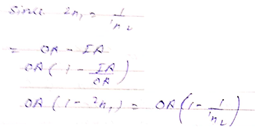Post By : Nek Singh Yadav 02 May, 2020 414 views Physics